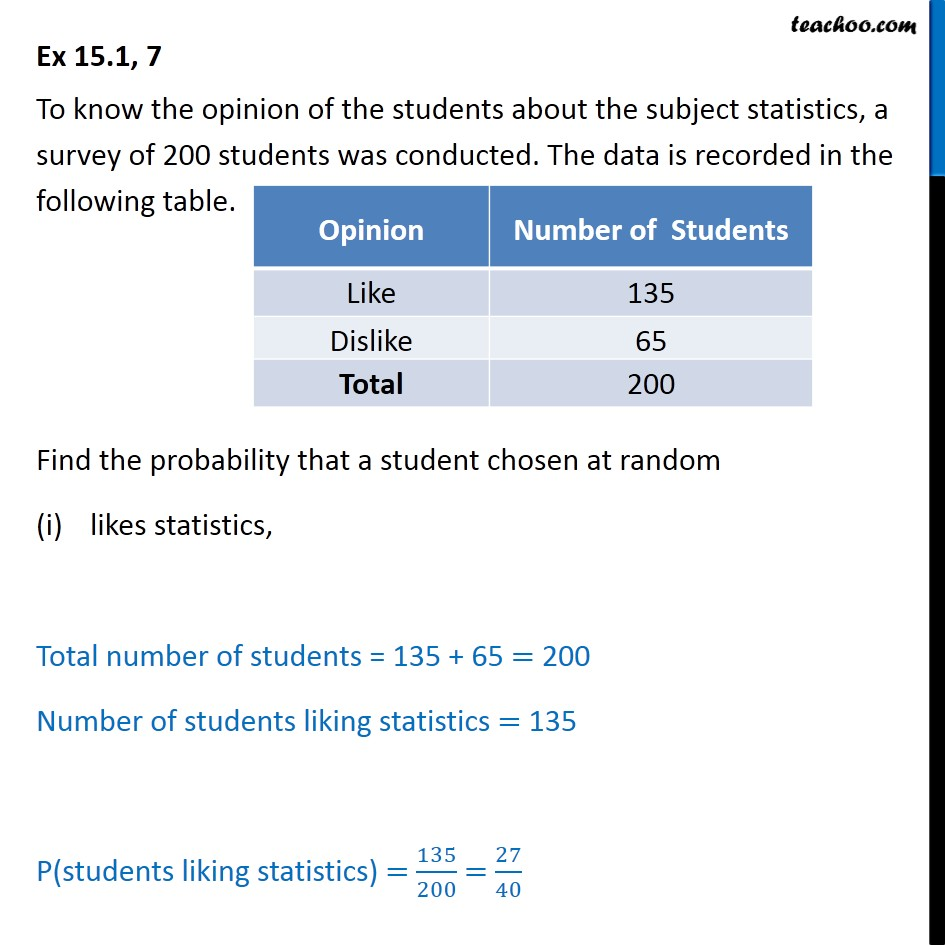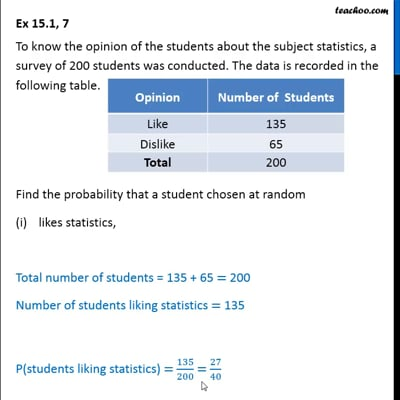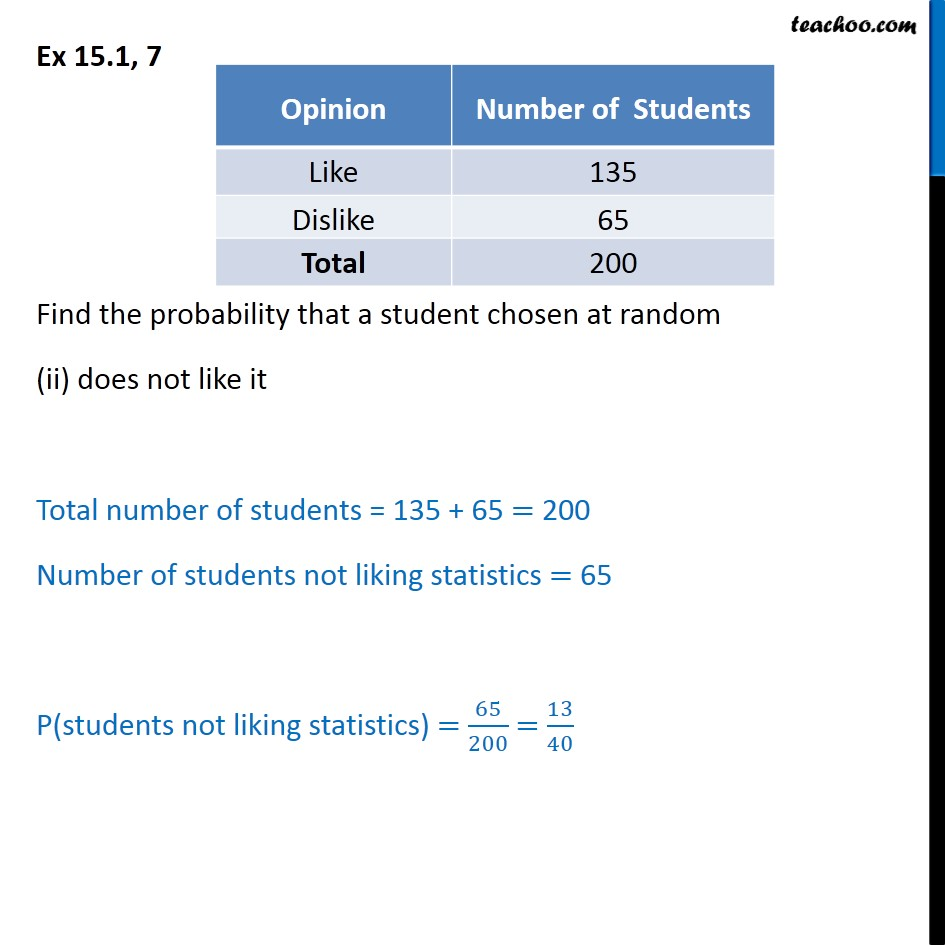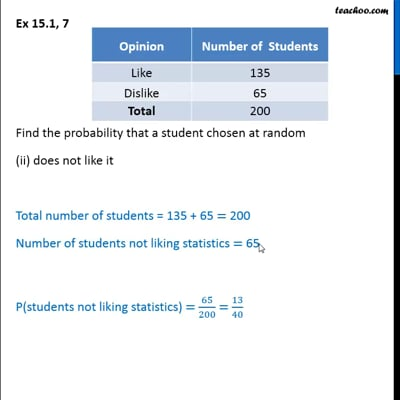Ex 15.1

Chapter 15 Class 9 Probability (Term 2)
Serial order wiseThis video is only available for Teachoo black usersThis video is only available for Teachoo black users

### Transcript

Ex 15.1, 7 To know the opinion of the students about the subject statistics, a survey of 200 students was conducted. The data is recorded in the following table. Find the probability that a student chosen at random likes statistics, Total number of students = 135 + 65 = 200 Number of students liking statistics = 135 P(students liking statistics) = 135/200 = 27/40 Ex 15.1, 7 Find the probability that a student chosen at random (ii) does not like it Total number of students = 135 + 65 = 200 Number of students not liking statistics = 65 P(students not liking statistics) = 65/200 = 13/40